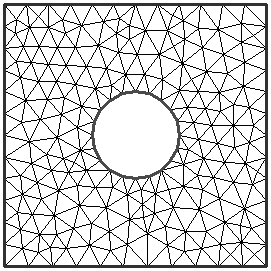# Uniform Mesh

In the Mesh Setup dialog, if the Mesh Type = Uniform, this will produce a mesh of approximately equally sized finite elements.

When the Mesh Type = Uniform, you will be required to specify the Element Type and the Approximate Number of Elements, in the Mesh Setup dialog.

In the example below:

• Mesh Type = Uniform
• Approximate Number of Elements = 300
• Element Type = Triangles

Uniform Mesh example## Approximate Number of Elements

For a Uniform mesh, an Approximate Number of Elements is entered, rather than a Gradation Factor and Number of Nodes, because:

• The Gradation Factor is by definition equal to 1 for a Uniform mesh.
• It is more intuitive to specify a total number of elements, rather than the number of boundary nodes, for a Uniform Mesh.

Remember that the Approximate Number of Elements is an approximate number. The actual number of elements generated is displayed in the status bar after meshing, and will in general be different from the Approximate Number of Elements entered in the Mesh Setup dialog.